# MCQ on Pie Chart

## MCQ on Pie Chart

Q1. Direction∶ The given pie chart depicts the expenditure incurred in crores towards each sport.

In the given pie-chart, what will be the central angle of the sector representing football?

1. 51°
2. 61.2°
3. 72.2°
4. 24°

#### Answer (Detailed Solution Below)

Option 2 : 61.2°

Q2. Study the pie chart that shows the quantity wise sales distribution of five products (A, B, C, D and E) of a company in 2019 and answer the question that follows.Quantity wise sales distribution of five products

(A. B. C. D and E)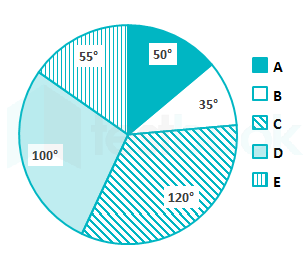If 2100 units of product C were sold in 2019 and the total number of units sold by the company in 2020 was 28% more than that in 2019, then how many units were sold by the company in 2020?

1. 8064
2. 8576
3. 6300
4. 7428

Answer: Option 1 : 8064

Q3. The pie chart shows weight in gms of ingredients required to bake a 90 gm cake. Study the diagram and answer the following questions.

If 2 dozen cakes each of 180 gms have to be baked, then how much butter (in gms) will be required?

1. 480
2. 240
3. 120
4. 360

Answer: Option 1 : 480

Q. The given pie charts represent the distribution of candidates who enrolled for a bank clerical examination and the candidates (out of those enrolled) who passed the examination, from five different institutes P, Q, R, S and T. Study the pie charts and answer the question that follows.

i. Total number of candidates who enrolled for the examination from five institutes = 5500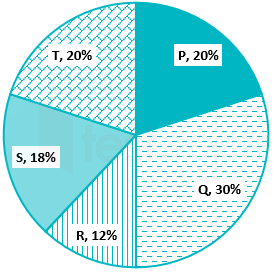ii. Total number of candidates who passed the examination from five institutes = 3300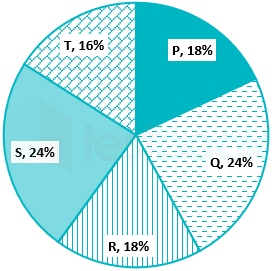The average number of candidates passed from institutes P and Q together is what percentage of the total number of candidates enrolled from institutes P and Q together?

1. 30%
2. 25.2%
3. 25.8%
4. 24.6%

Answer: Option 2 : 25.2%

### Q. Direction: Answer the question after studying the following pie chart.

This pie chart shows the symbolic monthly budget of a family with a monthly income of ₹36,000.

### The family spent Rs. 3,800 on the telephone bill in the current month. Suppose all other expenses are according to the budget, then how much rupees did the family save/invest in this current month?

1. Rs. 2,000
2. Rs. 1,200
3. Rs. 2,800
4. Rs. 7,200

#### Answer: Option 2 : Rs. 1,200

Q. The pie chart given below shows the annual snowfall received by 7 states of a country. The snowfall is shown as a percentage of total annual snowfall of the country.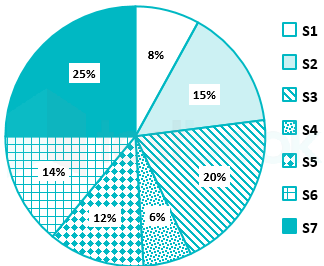If the annual snowfall received by the country is 700 cm. What is the difference in the annual snow falls of S3 and S2?

1. 42 cm
2. 7 cm
3. Cannot be determined
4. 35 cm

#### Answer: Option 4 : 35 cm

Q. Direction: Study the following pie-chart and answer the questions based on it.

1. 35%
2. 25%
3. 15%
4. 20%

Option 2 : 25%

### If the total amount spent on construction of house is Rs. 1 crore, find the excess amount spent on cement as compared to steel

1. Rs. 20 lacs
2. Rs. 1 lac
3. Rs. 2 lacs
4. Rs. 10 lacs

#### Answer (Detailed Solution Below)

Option 3 : Rs. 2 lacs

Q. The pie – chart shows percentage – wise distribution of teachers who teach six different subjects. Study the pie chart and answer the question: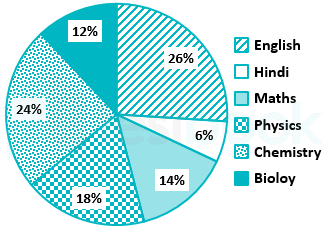Total number of teachers = 1650What is the total number of teachers teaching English, Maths and Physics?

1. 857
2. 950
3. 975
4. 957

#### Answer (Detailed Solution Below)

Option 4 : 957

Q. The following pie charts represent the distribution of candidates who were enrolled for a competitive examination, and the candidates (out of those enrolled) who passed the exam from five different institutes P,Q, R, S and T.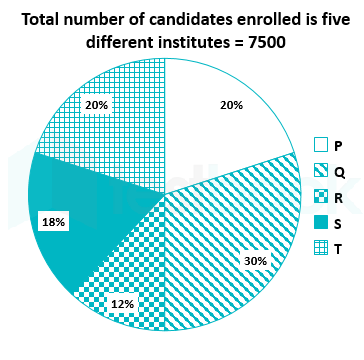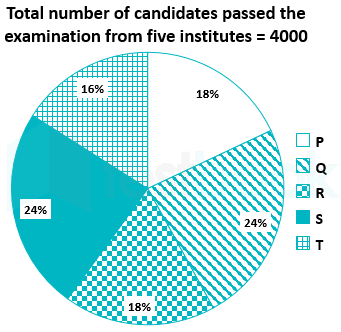What is the ratio of the total number of candidates enrolled in institutes Q, R and S together, to the number of candidates passed from the institutes Q, R and S together?

1. 15 : 71
2. 75 : 44
3. 71 : 15
4. 44 : 75

#### Answer (Detailed Solution Below)

Option 2 : 75 : 44

### Q. Comprehension:

Directions: The given pie chart shows information about the mobile phone manufacturing companies in India. The total number of mobile phone units manufactured is 12,40,000.

### How many units of mobile phones were manufactured by company ‘LMN’?

1. 3,72,000
2. 6,20,000
3. 12,40,000
4. 6,12,000

#### Answer (Detailed Solution Below)

Option 1 : 3,72,000

### Q. Comprehension:

The pie chart given below shows the number of students in 5 sections. The number of students is 7200.

1. 3060
2. 2900
3. 5300
4. 6000

Option 1 : 3060

### Q. Study the given pie chart and answer the question that follows.Distribution (degree wise) of employees working in offices A, B, C, D and E of a company in 2019.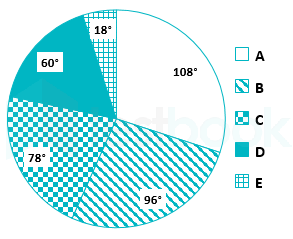Total Number of Employees = 300In which office is the number of employees approximately 18% more than the average number of employees working in offices A, C, D and E ?

1. D
2. A
3. B
4. C

#### Answer (Detailed Solution Below)

Option 4 : C

Q. Comprehension:

The following Pie-diagram shows the cost of construction of a house in Delhi. Study the diagram carefully and answer the questions.

1. 10
2. 5
3. 2
4. 3

Option 2 : 5

### Q. The pie chart shows the angular representation of five different Employment sectors. The total number of employees in these five sectors is 7,20,000.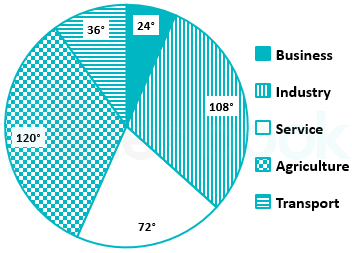How many total employees are engaged in Agriculture, Business and Transport?

1. 3,60,000
2. 5,00,000
3. 2,60,000
4. 4,80,000

#### Answer (Detailed Solution Below)

Option 1 : 3,60,000

## Q. Comprehension:

The pie chart given below shows the number of students in 5 sections. The number of students is 7200.

### The number of students in section A is what percent of that of section B?

1. 90
2. 110
3. 109
4. 111.11

#### Answer (Detailed Solution Below)

Option 4 : 111.11

Q. The number of students taking admission in First Year of a Degree Course at a college in areas of Social Sciences in 2012 was as follows: Economics (S1) – 96, Psychology (S2) – 84, History (S3) – 160. Political Science (S4) – 120. Sociology (S5) – 125. The break-up of students in different subjects has been presented through the given pie chart.Study the pie chart and answer the question that follows.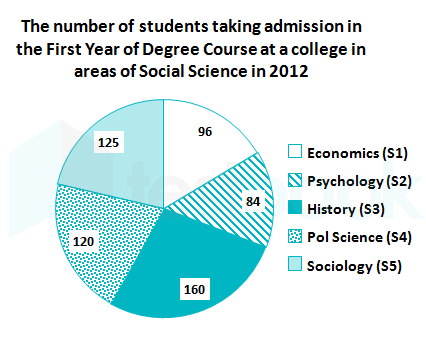In 2013, 20% of the Sociology students, 25% of the Political Science students, and one-sixth of the Economics students shifted to another college, and the number of History students got increased by 10% due to students shifting from other colleges. Over and above this, 10% of the remaining Sociology students and one-seventh of the Psychology students got transferred to Economics. What will be the central angle (to the nearest degree) of the sector corresponding to History in the pie chart, representing the break-up in 2013?

1. 120
2. 102
3. 126
4. 112

#### Answer (Detailed Solution Below)

Option 1 : 120

Q. Comprehension:

The pie chart given below shows the number of students in 5 sections. The number of students is 7200.

1. 1860
2. 1170
3. 1600
4. 1730

Option 2 : 1170

## Q. In the pie chart, the cost of construction of a house is shown (in degrees). Find the excess expenditure on bricks relative to the amount spent on iron, when the total cost of manufacture is Rs. 2,70,000.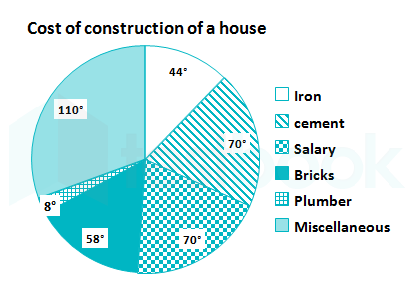1. Rs. 10500
2. Rs. 9000
3. Rs. 37500
4. Rs. 7650

#### Answer (Detailed Solution Below)

Option 1 : Rs. 10500

1. 80
2. 67
3. 60
4. 87

Option 4 : 87